• Python numpy 浮点数精度问题 在复现FP(fictitious play,Iterative solution of games by fictitious play-page393)算法的时候，迭代到中间发现没法复现paper里的结果，发现是numpy矩阵运算浮点数精度的问题。 ...
Python numpy 浮点数精度问题
在复现FP(fictitious play, Iterative solution of games by fictitious play-page393)算法的时候，迭代到中间发现没法复现paper里的结果，发现是numpy矩阵运算浮点数精度的问题。
具体问题
矩阵和向量相乘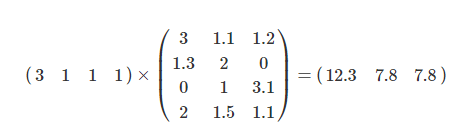然后取argmin想得到第一个7.8的index，也就是1。但由于精度的问题，导致两个7.8实际不一样大，取到了第二个7.8的index。 具体问题代码为
import numpy as np
x = np.matrix([3,1,1,1])*np.matrix([[3,1.1,1.2],[1.3,2,0],[0,1,3.1],[2,1.5,1.1]])
print('matrix: ',x)
print('value: ',x[0,0],x[0,1],x[0,2])
print('index: ',np.argmin(x))

得到
matrix:  [[12.3  7.8  7.8]]
value:  12.3 7.800000000000001 7.799999999999999
index:  2

可以发现明明相同的两个7.8由于精度变成了两个大小不同的数，所以argmin得到了2。
解决办法
二进制固有的问题，只能自己手动近似，用保留小数点位数消除误差。 如这里保留5位小数：
import numpy as np
x = np.round(np.matrix([3,1,1,1])*np.matrix([[3,1.1,1.2],[1.3,2,0],[0,1,3.1],[2,1.5,1.1]]),5)
print('matrix: ',x)
print('value: ',x[0,0],x[0,1],x[0,2])
print('index: ',np.argmin(x))

得到
matrix:  [[12.3  7.8  7.8]]
value:  12.3 7.8 7.8
index:  1

注意事项
这个办法不能解决所有问题，毕竟每个问题精度要求不一样。但由于计算机二进制的原因，没法从根本上解决，只能通过近似的方式，具体问题具体解决。
展开全文• 回归正题，博主在numpy中求一个整数矩阵A 的逆矩阵 结果A-1是float型， A点积A-1的结果也是浮点型，并不是好看的对角为1的矩阵 在此可以在初始设置 numpy.set_printoptions（suppress=True） %设置suppress...
引言，今年是py加入全国计算机二级大礼包的第一年，看了几道题，比如：
0.1+0.1==0.2  返回True
0.1+0.2==0.3返回False
都是0 1 二进制惹的祸，真是一点不省油。。。。弄好久   累
对于十进制，它只能表示以进制数的质因子为分母的分数。10 的质因子有 2 和 5。比如1/2、1/4、1/5、1/8和 1/10 都可以精确表示，因为这些分母只使用了10的质因子。相反，1/3、1/6 和 1/7 都是循环小数，因为它们的分母使用了质因子 3 或者 7。二进制下（进制数为2），只有一个质因子，即2。因此你只能精确表示分母质因子是2的分数。二进制中，1/2、1/4 和 1/8 都可以被精确表示。但是，1/5 或者 1/10 就变成了循环小数。所以，在十进制中能够精确表示的 0.1 与 0.2（1/10 与 1/5），到了计算机所使用的二进制数值系统中，就变成了循环小数。当你对这些循环小数进行数学运算时，并将二进制数据转换成人类可读的十进制数据时，会 对小数尾部进行截断处理。
不同语言运行0.1+0.2 可以见0.1+0.2在各种语言中的结果
回归正题，博主在numpy中求一个整数矩阵A 的逆矩阵 结果A-1是float型，  A点积A-1的结果也是浮点型，并不是好看的对角为1的矩阵
在此可以在初始设置
numpy.set_printoptions（suppress=True）
%设置suppress是不使用科学计数
%括号里也可以是precision=位数，小数的位数
例子
import numpy as np
from numpy.linalg import inv,qr
a=np.array([[0,1,2],[1,1,4],[2,-1,3]])%线性代数书上一个例子

inv(a)%求逆矩阵
Out:
array([[-7.,  5., -2.],
[-5.,  4., -2.],
[ 3., -2.,  1.]])
np.dot(a,inv(a))
Out:
array([[1.00000000e+00, 0.00000000e+00, 0.00000000e+00],
[0.00000000e+00, 1.00000000e+00, 4.44089210e-16],
[8.88178420e-16, 2.22044605e-16, 1.00000000e+00]])
%本应该是对角1矩阵的

np.set_printoptions(suppress=True)
np.dot(a,inv(a))

Out:
array([[1., 0., 0.],
[0., 1., 0.],
[0., 0., 1.]])
%现在就和py数据分析第二版里面的显示一样了


展开全文python
• python里直接导入decimal指定浮点数精度就可以解决。虽然有时候存在误处理状态。 import decimal decimal.getcontext().prec = 2 float(decimal.Decimal.from_float(2.9)/ decimal.Decimal.from_float(100)) ...
numpy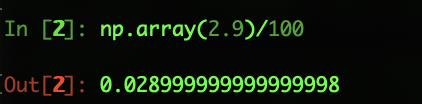elixir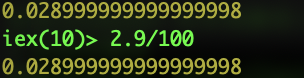python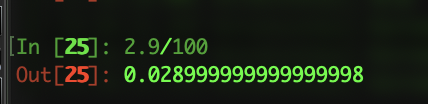clojure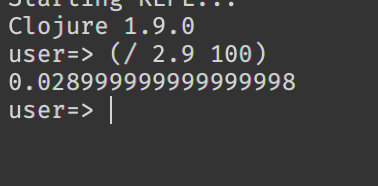因为这个问题不是计算错误，二是计算机浮点数除法的机制问题 python里直接导入decimal指定浮点数精度就可以解决。虽然有时候存在误处理状态。
import decimal
decimal.getcontext().prec = 2
float(decimal.Decimal.from_float(2.9)/ decimal.Decimal.from_float(100))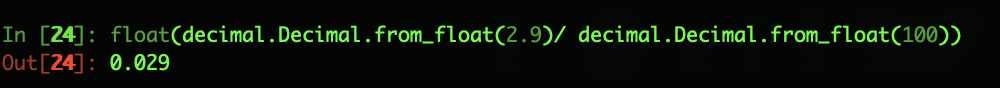符号计算也可以解决精度问题
import sympy
x , y = sympy.symbols('x y')
expr = x/y
expr.evalf(9/1)
expr.evalf(subs={x:2.9,y:100})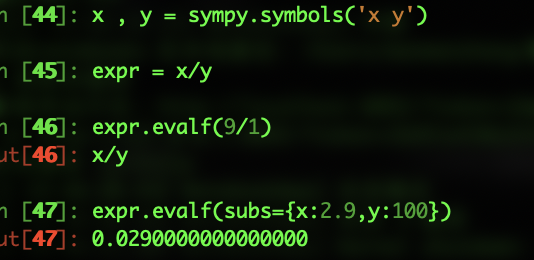展开全文• Let's say I have some 32-bit numbers and some 64-bit numbers:>... import numpy as np>>> w = np.float32(2.4)>>> x = np.float32(4.555555555555555)>>> y = np.float64(2....Let's say I have some 32-bit numbers and some 64-bit numbers:
>>> import numpy as np
>>> w = np.float32(2.4)
>>> x = np.float32(4.555555555555555)
>>> y = np.float64(2.4)
>>> z = np.float64(4.555555555555555)
I can print them out with %f but it has extra, unneeded decimals:
>>> '%f %f %f %f' % (w, x, y, z)
'2.400000 4.555555 2.400000 4.555556'
I can use %g but it seems to have a small default precision:
>>> '%g %g %g %g' % (w, x, y, z)
'2.4 4.55556 2.4 4.55556'
I was thinking I should use something like .7 for 32-bit values and .15 for 64-bit values:
>>> '%.7g %.7g %.15g %.15g' % (w, x, y, z)
'2.4 4.555555 2.4 4.55555555555556'
This seems to work reasonably well, but the precision number is also used up for numbers in front of the decimal place too, e.g. 34567.375768.
In summary, what is the correct way to serialize floating-point values to text such that it preserves appropriate precision for 32-bit and 64-bit values but doesn't use any unnecessary space?
Update:
Examples of what I think the output should be:
number float32 float64
5 5 5
0.1 0.1 0.1
2.4 2.4 2.4
4.555555555555555 4.5555553 4.5555555555555554
12345678.92345678635 12345679.0 12345678.923456786
What I get with .7/.16. This actually looks okay:
>>> v32 = np.array([5, 0.1, 2.4, 4.555555555555555, 12345678.92345678635], dtype=np.float32)
>>> v64 = np.array([5, 0.1, 2.4, 4.555555555555555, 12345678.92345678635], dtype=np.float64)
>>> ('%.7g ' * len(v32)) % tuple(v32)
'5 0.1 2.4 4.555555 1.234568e+07 '
>>> ('%.16g ' * len(v64)) % tuple(v64)
'5 0.1 2.4 4.555555555555555 12345678.92345679 '
解决方案
You could try to use the np.finfo function to get the precision corresponding to your float
finfo32 = np.finfo(np.float32)
finfo64 = np.finfo(np.float64)
finfo32.resolution = 1e-6
finfo64.resolution = 1e-15
Now that you know how many decimals you want, say, 6, just use a rstrip("0") to get rid of the unnecessary 0s:
print ("%.6f" % your_float).strip("0")
If you're leaning towards %g, perhaps you may want to use a dynamic format such as:
>>> strf = lambda v: ("%%.%ig" % max(np.ceil(np.log10(v)), 7)) % v
>>> strf(123.456789)
'123.45679'
>>> strf(123456789.12345)
'123456789'

展开全文• import numpy as np &gt;&gt;&gt; t1 = np.arange(0, 0.01*57, 0.01) &gt;&gt;&gt; t1.shape (58L,) 但是如果用同样的方法，可以得到正确的长度为58的、从0开始的、...python arange decimal
• 要想精确计算可以使用Decimal库,就可以避免这种情况了 from decimal import Decimal a = Decimal(‘1’) b = Decimal(‘0.2’) print(a+b+b+b)python
• A. 用Python计算9.4 - 9得到的结果为0.40000000000000036。 B. 结果用32位浮点数表示为： ...本人的计算机中存储的浮点数采用的是双精度浮点数，那么 +9.4转换为二进制即23×1.00101100110（0110循环），用64位浮python
• python中，浮点数运算，经常会碰到如下情况:? 出现上面的情况，主要还是因浮点数在计算机中实际是以二进制保存的，有些数不精确。 比如说: 0.1是十进制，转化为二进制后它是个无限循环的数：0....
• ## numpy设置输出精度

万次阅读 2018-03-05 13:48:22
使用set_printoptions设置输出的精度import numpy as np x=np.random.random(10) print(x) # [ 0.07837821 0.48002108 0.41274116 0.82993414 0.77610352 0.1023732 # 0.51303098 0.4617183 0.33487207 0.71162095.....
• 思路是先用numpy将待转换浮点数变为半精度存储，之后用tobytes()将内存里存储的二进制码转化为bytes类型返回，使用unpack解包为无符号短整型 最后格式化输出。 代码如下： import struct import numpy as np #转半...python modbus
• 最近在处理一个存档文件，用的两个字节来保存浮点数，不能理解，最后找到了这么一种数据类型：半精度浮点数 Python原生不支持这样的东西，需要外挂numpy，方可将双字节HEX转为半精度浮点 如果是字符串类型的“3c...buffer python
• numpy数组使用时发现了浮点数转整数可能会产生错误的问题，目前采用引入误差方式解决。 1、问题代码： e = 0 points = np.array([[100.10, 40.20], [100.30, 40.60], [100.20, 40.70]]) lons = points[:, 0] ...
• Python和Numpy中的整数类型是不一样的。它们之间是不能画等号的。python
• 当前，这是numpy支持的唯一扩展精度浮点类型。在x86-32和x86-64上，这是一种80位浮点类型。在更特殊的系统上，可能还有别的东西（在Sparc上的IIRC是实际的128位IEEE浮点数，在PPC上是double-double）。（这也可能取...
• 如果程序需要精确控制区间和数字精度，可以考虑使用numpy扩展库。Python 3.X对于浮点数默认的是提供17位数字的精度。关于单精度和双精度的通俗解释：单精度型和双精度型，其类型说明符为float 单精...
• python numpy的transpose函数用法 #MXNET的N*C*H*W在numpy打印时比较直观#mxnet卷积层# 输入数据格式是:batch * inchannel * height * width# 输出数据格式是:batch * ou ... JavaSwing程序设计（目录） 一.Java...
• ## Numpy

2020-02-14 21:32:43
4.1 Numpy优势 1 Numpy介绍 Numpy（Numerical Python）是一个开源的Python科学计算库，用于快速处理任意维度的数组。 Numpy支持常见的数组和矩阵操作。对于同样的数值计算任务，使用Numpy比直接使用Python要...
• ## numpy

2020-02-09 09:11:18
如果你使用 Python 语言进行科学计算，那么一定会接触到 NumPyNumPy 是支持 Python 语言的数值计算扩充库，其拥有强大的多维数组处理与矩阵运算能力。除此之外，NumPy 还内建了大量的函数，方便你快速构建数学模型...python 数据分析...